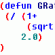Draw Rectangle Dimension

Recommended PostsHello! I was wondering how I would go about writing a function that would calculate the length of a diagonal of a rectangle using SQRT function? I've been trying but so far nothing!

Thanks!

Share on other sitesCan you post the code you wrote so far?

Presuming that you know the length, respectively width of the rectangle, you should use Pythagoras formula:

`(setq sizeDiagonal (sqrt (+ (expt sizeLength 2.0) (expt sizeWidth 2.0))))`

Share on other sitesThanks! I had a few simple lines, but I erased those as yours was basically what I needed. Would it be possible to write a function that would find the length & width itself? This one is out of curiosity.

Share on other sitesThanks! I had a few simple lines, but I erased those as yours was basically what I needed. Would it be possible to write a function that would find the length & width itself? This one is out of curiosity.

Try this:

```(defun LM:MAssoc ( key lst / item )
(if (setq item (assoc key lst))
(cons (cdr item) (LM:MAssoc key (cdr (member item lst))))
)
)
(defun c:getdist (/ s pt1 pt2 pt3 pt4 x y ent lst)
(setq s (ssget "_+.:E:S" '((0 . "LWPOLYLINE"))))
(if (= s nil)
(progn
(setq ent  (entget(ssname s 0)))
(setq lst (LM:MAssoc 10 ent))
(princ)
(setq pt1 (nth 0 lst))
(setq pt2 (nth 1 lst))
(setq pt3 (nth 2 lst))
(setq pt4 (nth 3 lst))
(setq x(distance pt1 pt2))
(setq y(distance pt1 pt4))
(princ (strcat "\nHeight: " (rtos y) "\nWidth: " (rtos x)))
(princ)
)
)
)
```

Yes, thanks to Lee Mac haha

Edit: But there is some problem with this code, if you rotate your rectangle by 90º the height will become width and vice versa.

Nothing that a if can't solve hehe

Edited by fabriciorby

Share on other sitesHere is another method:

```(defun c:test ( / lst sel )
(if (setq sel (ssget "_+.:E:S" '((0 . "LWPOLYLINE"))))
(progn
(setq lst
(mapcar 'cdr
(vl-remove-if-not '(lambda ( x ) (= 10 (car x)))
(entget (ssname sel 0))
)
)
)
(apply 'mapcar
(cons '-
(mapcar
'(lambda ( x ) (apply 'mapcar (cons x lst)))
'(max min)
)
)
)
)
)
)```

Join the conversation

You can post now and register later. If you have an account, sign in now to post with your account.
Note: Your post will require moderator approval before it will be visible.×   Pasted as rich text.   Paste as plain text instead

Only 75 emoji are allowed.